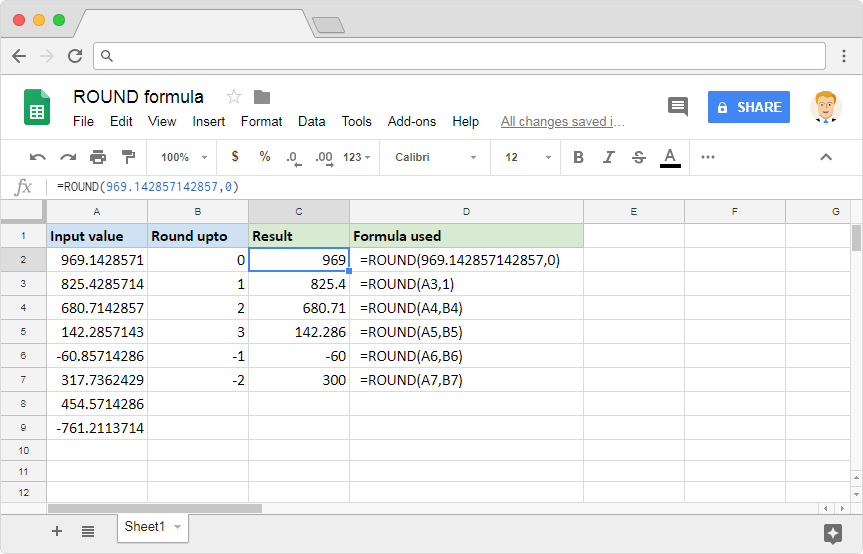# How to use the ROUND formula in Google SheetsWhile working with spreadsheets, we tend to come across many numbers that are rational. Some of them have more decimal values after the decimal point than desired. And our requirement may be such that we need to confine the rational values to the desired number of decimal places. We can do that with the help of the ROUND formula in Google Sheets.

### Syntax

ROUND(value, [places])

• value – is the numeric value that we need to round off. It can also be a reference to the cell that contains the numeric value.
• places – [ OPTIONAL – 0 by default ] – is the number of decimal places until which we need to round the value to.

Please note that the places parameter can also be negative. In such cases, the values are rounded at the specified number of digits to the left of the decimal point.

### Usage: ROUND formula in Google Sheets

Let’s go right ahead and try the formula on the Google Sheets application. This should reinforce our understanding of the formulas. Please consider the following screenshot.As is the case with almost all the Google Sheets formulas, both parameters of the ROUND formula accepts direct numeric values as well as cell references.

The very first example uses 0 for the places parameter. This indicates that the formula should ignore all the values after the decimal point, and then round off the number as per the standard norms. The values 1, 2, and 3 for places parameter results in one, two, and three values after the decimal point. We illustrate this in the examples in rows 3, 4 and 5.

It gets interesting when we start using the negative values for the places parameter. A value of -1 for the places parameter gets us one zero at the end, after rounding off the tenth place. But, there won’t be any decimal point in the output. Similarly, a value of -2 on the second parameter gets us two zeros at the end, after rounding off the hundredth place.